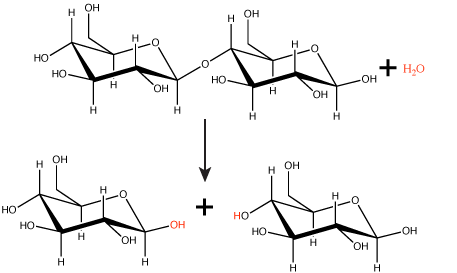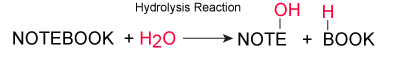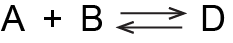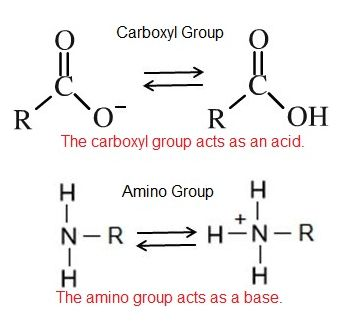# 2020_Spring_Bis2a_Facciotti_Lecture_04

### Learning Objectives Associated with 2020_Spring_Bis2a_Facciotti_Lecture_04

• Define the law of conservation of mass
• Explain the role of water in condensation reactions and hydrolytic reactions.
• Describe the equilibrium state.
• Discuss the concept of reaction reversibility and its relationship to equilibrium
• Relate the magnitude of the equilibrium constant to the equilibrium position
• Explain the effect of concentration change on a system at equilibrium
• Apply the concept of chemical equilibrium and the equilibrium constant to describe the progress of a chemical reaction, initially out of equilibrium, towards equilibrium, and finally at equilibrium in terms of “forward” and “reverse” reaction rates and concentrations of chemical reactants.
• Define pH and understand the relationship between pH and the hydrogen ion concentration.
• Use the definition of pH to determine the difference in [H+] concentration between two aqueous solutions

##Characteristic Chemical Reactions

Chemical reactions occur when two or more atoms bond together to form molecules or when bonded atoms break apart. We call the substances that "go in" to a chemical reaction reactants (by convention, we usually list these on the left side of a chemical equation), and the substances found that "come out" of the reaction products (by convention, we usually list these on the right side of a chemical equation). An arrow drawn between reactants and products typically shows the direction of the chemical reaction. By convention, for one-way reactions (a.k.a. unidirectional), reactants are listed on the left and products on the right of the single-headed arrow. However, you should be able to identify reactants and products of unidirectional reactions that are written in any orientation (e.g. right-to-left; top-to-bottom, diagonal right-to-left, around a circular arrow, etc.) by using the arrow to orient yourself.

In chemical reactions, the atoms and elements present in the reactant(s) must all also be present in the product(s). Similarly, there can be nothing present in the products that was not present in the reactants. This is because chemical reactions are governed by the law of conservation of mass, which states that matter cannot be created nor destroyed in a chemical reaction. This means that when you examine a chemical reaction, you must try to account for everything that goes in AND make sure that you can find it all in the stuff that comes out!

Just as you can express mathematical calculations in equations such as 2 + 7 = 9, you can use chemical equations to show how reactants become products. By convention, chemical equations are typically read or written from left to right. Reactants on the left are separated from products on the right by a single- or double-headed arrow indicating the direction in which the chemical reaction proceeds. For example, the chemical reaction in which one atom of nitrogen and three atoms of hydrogen produce ammonia would be written as:

$\ce{N + 3H→NH_3}.$

Correspondingly, the breakdown of ammonia into its components would be written as:

$\ce{NH3→N + 3H.}$

Note that in either direction, you find 1 N and 3 Hs on both sides of the equation.

Possible NB DiscussionPoint

In BIS 2A, it is important to appreciate the law of the conservation of mass in the context of biological processes.  In chemistry, you will take a quantitative approach to this topic, learning to balance equations, and making sure that the total number of atoms and the total charge does not change.  In BIS2A, we take a more qualitative approach to the topic. Do you think this leads to confusion? Should we place more emphasis on balancing equations in BIS2A?

### Reversibility

While all chemical reactions can technically proceed in both directions, some reactions tend to favor one direction over the other. Depending on the degree to which a reaction spontaneously proceed in either both or one direction a different name can be given to characterize the reactions reversibility. Some chemical reactions, such as the one shown above, proceed mostly in one direction with the "reverse" direction happening on such long time scales or with such low probability that, for practical purposes, we ignore the "reverse" reaction. These unidirectional reactions are also called irreversible reactions and are depicted with a single-headed (unidirectional) arrow. By contrast, reversible reactions are those that can readily proceed in either direction. Reversible reactions are usually depicted by a chemical equation with a double-headed arrow pointing toward both the reactants and the products. In practice, you will find a continuum of chemical reactions; some proceed mostly in one direction and nearly never reverse, while others change direction easily depending on various factors like the relative concentrations of reactants and products. These terms are just ways of describing reactions with different equilibrium points.

Use of vocabulary

You may have realized that the terms "reactants" and "products" are relative to the direction of the reaction. If you have a reaction that is reversible, though, the products of running the reaction in one direction become the reactants of the reverse. You can label the same compound with two different terms. That can be a bit confusing. So, what is one to do in such cases? The answer is that if you want to use the terms "reactants" and "products", you must be clear about the direction of reaction that you are referring to - even for when discussing reversible reactions. The choice of terms, "reactants" or "products" that you use will communicate to others the directionality of the reaction that you are considering.

Let's look at an example of a reversible reaction in biology and discuss an important extension of these core ideas that arises in a biological system. In human blood, excess hydrogen ions (H+) bind to bicarbonate ions (HCO3-), forming an equilibrium state with carbonic acid (H2CO3). This reaction is readily reversible. If carbonic acid were added to this system, some of it would be converted to bicarbonate and hydrogen ions as the chemical system sought out equilibrium.

$\ce{HCO_3^−+ H^+\rightleftharpoons H_2CO_3}$

The example above examines and "idealized" chemical systems as it might occur in a test-tube. In biological systems, however, equilibrium for a single reaction is rarely reached as it might be in the test-tube. In biological systems, reactions do not occur in isolation. Rather, the concentrations of the reactants and/or products are constantly changing, often with a product of one reaction being a reactant for another reaction. These linked reactions form what are known as biochemical pathways. The immediate example below illustrates this point. While the reaction between the bicarbonate/proton and carbonic acid is highly reversible, it turns out that, physiologically, this reaction is usually "pulled" toward the formation of carbonic acid. Why? As shown below, carbonic acid becomes a reactant for another biochemical reactionthe conversion of carbonic acid to CO2 and H2O. This conversion reduces the concentration of H2CO3, thus pulling the reaction between bicarbonate and H+ to the right. Moreover, a third, unidirectional reaction, the removal of CO2 and H2O from the system, also pulls the reaction further to the right. These kinds of reactions are important contributors to maintaining the H+ homeostasis of our blood.

$\ce{HCO_3^- + H^+ \rightleftharpoons H_2CO_3 \rightleftharpoons CO_2 + H_20 \rightarrow} \text{ waste}$

The reaction involving the synthesis of carbonic acid is actually linked to its breakdown into $$CO_2$$ and $$H_2O$$. These products are then removed from the system/body when they are exhaled. Together, the breakdown of carbonic acid and the act of exhaling the products pull the first reaction to the right.

### Synthesis reactions

Many macromolecules are made from smaller subunits, or building blocks, called monomers. Monomers covalently link to form larger molecules known as polymers. Often, the synthesis of polymers from monomers will also produce water molecules as products of the reaction. This type of reaction is known as dehydration synthesis or condensation reaction.Figure 1. In the dehydration synthesis reaction depicted above, two molecules of glucose are linked together to form the disaccharide maltose. In the process, a water molecule is formed. Attribution: Marc T. Facciotti (original work)

Interactive Figure 1. The molecules of glucose and maltose depicted as 3D interactive molecules.

 Glucose Maltose

In a dehydration synthesis reaction (Figure 1), the hydrogen of one monomer combines with the hydroxyl group of another monomer, releasing a molecule of water. At the same time, the monomers share electrons and form covalent bonds. As additional monomers join, this chain of repeating monomers forms a polymer. Different types of monomers can combine in many configurations, giving rise to a diverse group of macromolecules. Even one kind of monomer can combine in a variety of ways to form several different polymers; for example, glucose monomers are the constituents of starch, glycogen, and cellulose.

In the carbohydrate monomer example above, the polymer is formed by a dehydration reaction; this type of reaction is also used to add amino acids to a growing peptide chain and nucleotides to the growing DNA or RNA polymer. Visit the modules on Amino Acids, Lipids, and Nucleic Acids to see if you can identify the water molecules that are removed when a monomer is added to the growing polymer.Figure 2. This depicts, using words, (decorated with functional groups colored in red) a generic dehydration synthesis/condensation reaction. Attribution: Marc T. Facciotti (original work)

### Hydrolysis reactions

Polymers are broken down into monomers in a reaction known as hydrolysis. A hydrolysis reaction includes a water molecule as a reactant (Figure 3). During these reactions, a polymer can be broken into two components: one product carries a hydrogen ion (H+) from the water, while the second product carries the water's remaining hydroxide (OH).Figure 3. In the hydrolysis reaction shown here, the disaccharide maltose is broken down to form two glucose monomers with the addition of a water molecule. Note that this reaction is the reverse of the synthesis reaction shown in Figure 1 above. Attribution: Marc T. Facciotti (original work)Figure 4. This depicts using words (decorated with functional groups colored in red) a generic hydrolysis reaction. Attribution: Marc T. Facciotti (original work)

Dehydration synthesis and hydrolysis reactions are catalyzed, or “sped up,” by specific enzymes. Note that both dehydration synthesis and hydrolysis reactions involve the making and breaking of bonds between the reactantsa reorganization of the bonds between the atoms in the reactants. In biological systems (our bodies included), food in the form of molecular polymers is hydrolyzed into smaller molecules by water via enzyme-catalyzed reactions in the digestive system. This allows for the smaller nutrients to be absorbed and reused for a variety of purposes. In the cell, monomers derived from food may then be reassembled into larger polymers that serve new functions.

Visit this site to see visual representations of dehydration synthesis and hydrolysis.
Example of Hydrolysis with Enzyme Action is shown in this 3 minute video entitled: Hydrolysis of Sucrose by Sucrase.

### Exchange/transfer reactions

We will also encounter reactions termed exchange reactions. In these types of reactions, "parts" of molecules are transferred between one anotherbonds are broken to release a part of a molecule and bonds are formed between the released part and another molecule. These enzyme-catalyzed reactions are usually reasonably complex multi-step chemical processes.Figure 5. An exchange reaction in which both synthesis and hydrolysis can occur, chemical bonds are both formed and broken, is depicted using a word analogy.

## Chemical equilibrium—Part 1: forward and reverse reactions

Understanding the concept of chemical equilibrium is critical to following several of the discussions that we have in BIS2A and indeed throughout biology and the sciences. It is difficult to completely describe the concept of chemical equilibrium without reference to the energy of a system, but for the sake of simplicity, let’s try anyway and reserve the discussion of energy for another chapter. Let us, rather, begin developing our understanding of equilibrium by considering the reversible reaction below:Hypothetical reaction #1: A hypothetical reaction involving compounds A, B and D. If we read this from left to right, we would say that A and B come together to form a larger compound: D. Reading the reaction from right to left, we would say that compound D breaks down into smaller compounds: A and B.

We first need to define what is meant by a “reversible reaction.” The term “reversible” simply means that a reaction can proceed in both directions. That is, the things on the left side of the reaction equation can react together to become the things on the right of the equation, AND the things on the right of the equation can also react together to become the things on the left side of the equation. Reactions that only proceed in one direction are called irreversible reactions.

To start our discussion of equilibrium, we begin by considering a reaction that we posit is readily reversible. In this case, it is the reaction depicted above: the imaginary formation of compound D from compounds A and B. Since it is a reversible reaction, we could also call it the decomposition of D into A and B. Let us, however, imagine an experiment in which we watch the reaction proceed from a starting point where only A and B are present.

### Example #1: Left-balanced reaction

Hypothetical reaction #1: time course
Concentration t=0 t=1 t=5 t=10 t=15 t=20 t=25 t=30 t=35 t=40
[A] 100 90 80 70 65 62 60 60 60 60
[B] 100 90 80 70 65 62 60 60 60 60
[C] 0 10 20 30 45 38 40 40 40 40

At time t = 0 (before the reaction starts), the reaction has 100 concentration units of compounds A and B and zero units of compound D. We now allow the reaction to proceed and observe the individual concentrations of the three compounds over time (t=1, 5, 10, 15, 20, 25, 30, 35, and 40 time units). As A and B react, D forms. In fact, one can see D forming from t=0 all the way to t=25. After that time, however, the concentrations of A, B and D stop changing. Once the reaction reaches the point where the concentrations of the components stop changing, we say that the reaction has reached equilibrium. Notice that the concentrations of A, B, and D are not equal at equilibrium. In fact, the reaction seems left balanced so that there is more A and B than D.

Note: Common student misconception warning

Many students fall victim to the misconception that the concentrations of a reaction’s reactants and products must be equal at equilibrium. Given that the term equilibrium sounds a lot like the word “equal,” this is not surprising. But as the experiment above tries to illustrate, this is NOT correct!

### Example #2: Right-balanced reaction

We can examine a second hypothetical reaction, the synthesis of compound $$\ce{J}$$ from the compounds $$\ce{E}$$ and $$\ce{F}$$.

$\ce{E +F <=> J} \nonumber$

Hypothetical reaction #2: A hypothetical reaction involving compounds E, F and J. If we read this from left to right, we would say that E and F come together to form a larger compound: J. Reading the reaction from right to left, we would say that compound J breaks down into smaller compounds: E and F.

The structure of hypothetical reaction #2 looks identical to that of hypothetical reaction #1, which we considered above—two things come together to make one bigger thing. We just need to assume, in this case, that E, F, and J have different properties from A, B, and D. Let’s imagine a similar experiment to the one described above and examine this data:

Hypothetical reaction #2: time courseIn this case, the reaction also reaches equilibrium. This time, however, equilibrium occurs at around t=30. After that point, the concentrations of E, F, and J do not change. Note again that the concentrations of $$\ce{E}$$, $$\ce{F}$$, and $$\ce{J}$$ are not equal at equilibrium. In contrast to hypothetical reaction #1 (the ABD reaction), this time the concentration of J, the thing on the right side of the arrows, is at a higher concentration than E and F. We say that, for this reaction, equilibrium lies to the right.

Four more points need to be made at this juncture.

• Point 1: Whether equilibrium for a reaction lies to the left or the right will be a function of the properties of the components of the reaction and the environmental conditions that the reaction is taking place in (e.g., temperature, pressure, etc.).
• Point 2: We can also talk about equilibrium using concepts of energy, and we will do this soon, just not yet.
• Point 3: While hypothetical reactions #1 and #2 appear to reach a point where the reaction has “stopped,” you should imagine that reactions are still happening even after equilibrium has been reached. At equilibrium the “forward” and “reverse” reactions are just happening at the same rate. That is, in example #2, at equilibrium J is forming from E and F at the same rate that it is breaking down into E and F. This explains how the concentrations of the compounds aren’t changing despite the fact that the reactions are still happening.
• Point 4: From this description of equilibrium, we can define something we call the equilibrium constant. Typically, the constant is represented by an uppercase K and may be written as Keq. In terms of concentrations, Keq is written as the mathematical product of the reaction product concentrations (stuff on the right) divided by the mathematical product of the reactant concentrations (stuff on the left). For example, Keq,1 = [D]/[A][B], and Keq,2 = [J]/[E][F]. The square brackets "[]" indicate the “concentration of” whatever is inside the bracket.

Possible NB DiscussionPoint

The following statement is true: A chemical equilibrium can be established starting with equal concentrations of reactants and products. Can you think of and describe other starting conditions for which a chemical equilibrium can also be established?  Are there any starting conditions for which a chemical equilibrium can NOT be established?

## What is the role of Acid/Base Chemistry in Bis2A?We have learned that the behavior of chemical functional groups depends greatly on the composition, order and properties of their constituent atoms. As we will see, some of the properties of key biological functional groups can be altered depending on the pH (hydrogen ion concentration) of the solution that they are bathed in.

For example, some of the functional groups on the amino acid molecules that make up proteins can exist in different chemical states depending on the pH. We will learn that the chemical state of these functional groups in the context of a protein can have have a profound effect on the shape of protein or its ability to carry out chemical reactions. As we move through the course we will see numerous examples of this type of chemistry in different contexts.

pH is formally defined as:

$pH = -\log_{10} [H^+]$

In the equation above, the square brackets surrounding $$H^+$$ indicate concentration. If necessary, try a math review at wiki logarithm or kahn logarithm. Also see: concentration dictionary or wiki concentration.

Hydrogen ions are spontaneously generated in pure water by the dissociation (ionization) of a small percentage of water molecules into equal numbers of hydrogen (H+) ions and hydroxide (OH-) ions. While the hydroxide ions are kept in solution by their hydrogen bonding with other water molecules, the hydrogen ions, consisting of naked protons, are immediately attracted to un-ionized water molecules, forming hydronium ions (H30+).

Still, by convention, scientists refer to hydrogen ions and their concentration as if they were free in this state in liquid water. This is another example of a shortcut that we often take - it's easier to write H+ rather than H3O+. We just need to realize that this shortcut is being taken; otherwise confusion will ensue.Figure 1: Water spontaneously dissociates into a proton and hydroxyl group. The proton will combine with a water molecule forming a hydronium ion.

The pH of a solution is a measure of the concentration of hydrogen ions in a solution (or the number of hydronium ions). The number of hydrogen ions is a direct measure of how acidic or how basic a solution is.

The pH scale is logarithmic and ranges from 0 to 14 (Figure 2). We define pH=7.0 as neutral. Anything with a pH below 7.0 is termed acidic and any reported pH above 7.0 is termed alkaline or basic. Extremes in pH in either direction from 7.0 are usually considered inhospitable to life, although examples exist to the contrary. pH levels in the human body usually range between 6.8 and 7.4, except in the stomach where the pH is more acidic, typically between 1 and 2.Figure 2: The pH scale ranging from acidic to basic with various biological compounds or substances that exist at that particular pH. Attribution: Marc T. Facciotti

Watch this video for an alternative explanation of pH and its logarithmic scale.

The concentration of hydrogen ions dissociating from pure water is 1 × 10-7 moles H+ ions per liter of water.

1 mole (mol) of a substance (which can be atoms, molecules, ions, etc), is defined as being equal to 6.02 x 1023 particles of the substance. Therefore, 1 mole of water is equal to 6.02 x 1023 water molecules. The pH is calculated as the negative of the base 10 logarithm of this unit of concentration. The log10 of 1 × 10-7 is -7.0, and the negative of this number yields a pH of 7.0, which is also known as neutral pH.

Non-neutral pH readings result from dissolving acids or bases in water. High concentrations of hydrogen ions yields a low pH number, whereas low levels of hydrogen ions result in a high pH.

This inverse relationship between pH and the concentration of protons confuses many students - take the time to convince yourself that you "get it."

An acid is a substance that increases the concentration of hydrogen ions (H+) in a solution, usually by having one of its hydrogen atoms dissociate. For example, we have learned that the carboxyl functional group is an acid. The hydrogen atom can dissociate from the oxygen atom resulting in a free proton and a negatively charged functional group. A base provides either hydroxide ions (OH) or other negatively charged ions that combine with hydrogen ions, effectively reducing the H+ concentration in the solution and thereby raising the pH. In cases where the base releases hydroxide ions, these ions bind to free hydrogen ions, generating new water molecules. For example, we have learned that the amine functional group is a base. The nitrogen atom will accept hydrogen ions in solution, thereby reducing the number of hydrogen ions which raises the pH of the solution.Figure 3: The carboxylic acid group acts as an acid by releasing a proton into solution. This increases the number of protons in solution and thus decreases the pH. The amino group acts as a base by accepting hydrogen ions from solution, decreasing the number of hydrogen ions in solutions, thus increasing the pH.